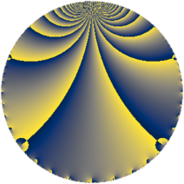# Properties

 Label 304.3.eLevel $304$ Weight $3$ Character orbit 304.e Rep. character $\chi_{304}(113,\cdot)$ Character field $\Q$ Dimension $19$ Newform subspaces $7$ Sturm bound $120$ Trace bound $5$

# Related objects

## Defining parameters

 Level: $$N$$ $$=$$ $$304 = 2^{4} \cdot 19$$ Weight: $$k$$ $$=$$ $$3$$ Character orbit: $$[\chi]$$ $$=$$ 304.e (of order $$2$$ and degree $$1$$) Character conductor: $$\operatorname{cond}(\chi)$$ $$=$$ $$19$$ Character field: $$\Q$$ Newform subspaces: $$7$$ Sturm bound: $$120$$ Trace bound: $$5$$ Distinguishing $$T_p$$: $$3$$, $$5$$

## Dimensions

The following table gives the dimensions of various subspaces of $$M_{3}(304, [\chi])$$.

Total New Old
Modular forms 86 21 65
Cusp forms 74 19 55
Eisenstein series 12 2 10

## Trace form

 $$19 q - 2 q^{5} - 14 q^{7} - 61 q^{9} + O(q^{10})$$ $$19 q - 2 q^{5} - 14 q^{7} - 61 q^{9} + 2 q^{11} - 26 q^{17} + 33 q^{19} + 26 q^{23} + 73 q^{25} - 44 q^{35} + 32 q^{39} - 126 q^{43} - 34 q^{45} - 94 q^{47} + 121 q^{49} - 116 q^{55} - 40 q^{57} - 82 q^{61} + 418 q^{63} + 54 q^{73} - 180 q^{77} + 403 q^{81} + 82 q^{83} - 244 q^{85} - 528 q^{87} - 80 q^{93} + 210 q^{95} + 82 q^{99} + O(q^{100})$$

## Decomposition of $$S_{3}^{\mathrm{new}}(304, [\chi])$$ into newform subspaces

Label Dim $A$ Field CM Traces $q$-expansion
$a_{2}$ $a_{3}$ $a_{5}$ $a_{7}$
304.3.e.a $1$ $8.283$ $$\Q$$ $$\Q(\sqrt{-19})$$ $$0$$ $$0$$ $$-9$$ $$5$$ $$q-9q^{5}+5q^{7}+9q^{9}-3q^{11}+15q^{17}+\cdots$$
304.3.e.b $2$ $8.283$ $$\Q(\sqrt{-29})$$ None $$0$$ $$0$$ $$-8$$ $$2$$ $$q+\beta q^{3}-4q^{5}+q^{7}-20q^{9}-14q^{11}+\cdots$$
304.3.e.c $2$ $8.283$ $$\Q(\sqrt{-2})$$ None $$0$$ $$0$$ $$-2$$ $$-10$$ $$q+\beta q^{3}-q^{5}-5q^{7}+q^{9}-5q^{11}+\cdots$$
304.3.e.d $2$ $8.283$ $$\Q(\sqrt{-13})$$ None $$0$$ $$0$$ $$8$$ $$10$$ $$q+\beta q^{3}+4q^{5}+5q^{7}-4q^{9}+10q^{11}+\cdots$$
304.3.e.e $2$ $8.283$ $$\Q(\sqrt{57})$$ $$\Q(\sqrt{-19})$$ $$0$$ $$0$$ $$9$$ $$-5$$ $$q+(5-\beta )q^{5}+(-1-3\beta )q^{7}+9q^{9}+\cdots$$
304.3.e.f $2$ $8.283$ $$\Q(\sqrt{-2})$$ None $$0$$ $$0$$ $$14$$ $$-22$$ $$q+\beta q^{3}+7q^{5}-11q^{7}-23q^{9}-3q^{11}+\cdots$$
304.3.e.g $8$ $8.283$ $$\mathbb{Q}[x]/(x^{8} + \cdots)$$ None $$0$$ $$0$$ $$-14$$ $$6$$ $$q+\beta _{1}q^{3}+(-2-\beta _{4})q^{5}+(1-\beta _{3})q^{7}+\cdots$$

## Decomposition of $$S_{3}^{\mathrm{old}}(304, [\chi])$$ into lower level spaces

$$S_{3}^{\mathrm{old}}(304, [\chi]) \cong$$ $$S_{3}^{\mathrm{new}}(19, [\chi])$$$$^{\oplus 5}$$$$\oplus$$$$S_{3}^{\mathrm{new}}(38, [\chi])$$$$^{\oplus 4}$$$$\oplus$$$$S_{3}^{\mathrm{new}}(76, [\chi])$$$$^{\oplus 3}$$$$\oplus$$$$S_{3}^{\mathrm{new}}(152, [\chi])$$$$^{\oplus 2}$$